Pay

## Communication diagram example

8
0
1
publish time: 2020-09-15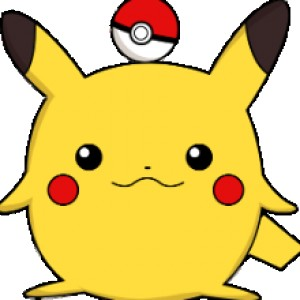easy diagrams

This is an example of a UML communication diagram.

See More Related TemplatesThis is an example of a UML diagram about adding events to the calendar.
UML Diagram - Add event to calendar
23
1
0easy diagrams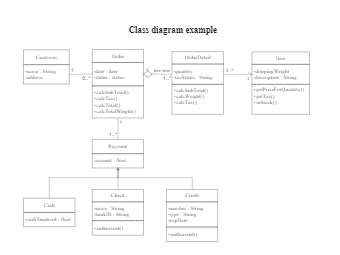This is an example of a uml class diagram.
Class diagram example
174
5
4easy diagrams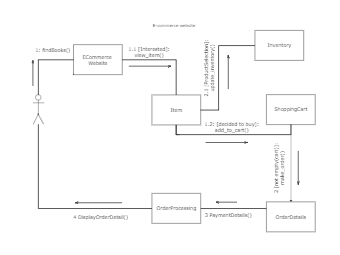This is an example of a UML collaboration diagram.
ecommerce website - UML diagram
14
1
0easy diagrams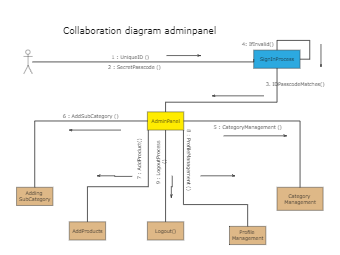This is an example of a UML collaboration diagram about adminpanel.
Collaboration diagram adminpanel
7
1
0easy diagrams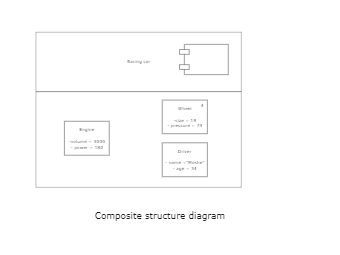This is an example of a composite structure diagram.
Composite structure diagram
3
0
0easy diagrams